在线英语听力室_免费在线英语听力学习网站

纪录片《重力错乱》 第04期 牛顿万有引力定律

(单词翻译:双击或拖选)

Newton believed that gravity is a force that acts throughout the entire universe.

In 1686, he finally managed to break it down into one single mathematical equation.

1686年，他终于设法将它分解到了一个简单的数学等式里了。

Newton's understanding of gravity is actually incredibly simple

that the force between two objects depends on only two things, the mass of the objects and the distance they are apart.

So, the more massive the objects, the stronger the force,

and, the further the objects are apart, the weaker the force.

See, it's easy to show actually.

Got a pen? So this is Newton's law of gravitation.

The force is equal to the masses of the two objects, divided by the square of the distance apart of the objects.

And then there's Newton's gravitational constant,

that just sets the scale -- it tells you the overall strength of gravity.

With one beautiful bit of maths, Newton had figured out gravity, but not just here on Earth.

The Moon seemed to orbit the Earth exactly as he predicted, as did the planets orbiting around the sun.

Newton believed we live in a universe in which ultimately the movement of everything can be predicted.

Newton's universal law of gravitation is one of the most important turning points in physics,

and that's because it really is universal.

It allows you to predict not only how things move under the influence of gravity here on Earth

but how the stars and planets and even galaxies1 move, all the way across the universe.

 1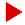星系( galaxy的名词复数 )； 银河系； 一群（杰出或著名的人物） 参考例句： Quasars are the highly energetic cores of distant galaxies. 类星体是遥远星系的极为活跃的核心体。 We still don't know how many galaxies there are in the universe. 我们还不知道宇宙中有多少个星系。

•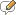评论
•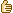推荐
• 收藏
• 挑错
• 打印
------分隔线----------------------------
TAG标签：   纪录片  重力错乱

(0)
0%

(0)
0%

•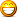•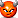•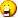•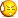•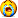•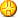•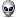﻿